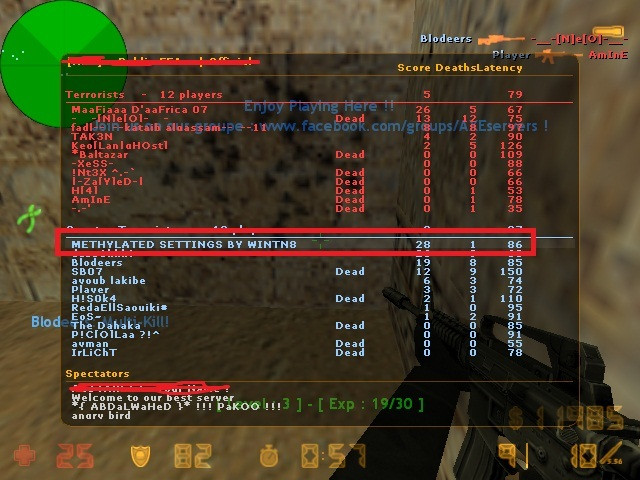02.07.2016, 22:13
Hello Guys , Those Settings are AMAZING AND LEGIT
Screenshot :

GUYS HIDE = 0 // PLEASE GIVE REPITIONS & THANKS

[http://rgho.st/8n2f4hgX9.view]Спойлер:
settings maded by wintn8...... legit settings for methylated
have a fun
legit_aimbot
legit_triggerbot

[Misc]
bhop = 0

draw_recoil = 0
draw_trigger = 0

aim_draw_pw = 0
aim_draw_spot = 1
aim_draw_spot_color = 255,0,255
aim_draw_fov = 0

antiscreen_time = 6

lambert = 0

[Keys]

toggle_hack = none
toggle_aim = none
toggle_esp = none
toggle_trigger = none
toggle_gui = none

key_trigger = none

[ESP]
esp = 0
esp_dm = 0
esp_health = 0
esp_name = 0
esp_wh_blocker = 0

[DEAGLE]
aim = 1
aim_auto = 0
aim_fov = 11
aim_smooth = 13
aim_wait = 3
aim_spot = 11
aim_use_bone = 0
aim_perfectwall = 0
aim_perfectwall_sens = 0

recoil = 0
recoil_value = 0

trigger = 1
trigger_dm = 0
trigger_aim = 0

[ELITE]
aim = 1
aim_auto = 0
aim_fov = 9
aim_smooth = 3.5
aim_wait = 0
aim_spot = 11
aim_use_bone = 0
aim_perfectwall = 0
aim_perfectwall_sens = 0

recoil = 0
recoil_value = 0

trigger = 1
trigger_dm = 0

[GALIL]
aim = 1
aim_auto = 0
aim_fov = 15
aim_smooth = 14
aim_wait = 0
aim_spot = 11
aim_use_bone = 0
aim_perfectwall = 1
aim_perfectwall_sens = 1

recoil = 1
recoil_value = 1

trigger = 1
trigger_dm = 0
trigger_aim = 0

[FAMAS]
aim = 1
aim_auto = 0
aim_fov = 11
aim_smooth = 13
aim_wait = 0
aim_spot = 11
aim_use_bone = 0
aim_perfectwall = 1
aim_perfectwall_sens = 1

recoil = 1
recoil_value = 1

trigger = 0
trigger_dm = 0
trigger_aim = 0

[FIVESEVEN]
aim = 0
aim_auto = 0
aim_fov = 0
aim_smooth = 0
aim_wait = 0
aim_spot = 0
aim_use_bone = 0
aim_perfectwall = 0
aim_perfectwall_sens = 0

recoil = 0
recoil_value = 0

trigger = 1
trigger_dm = 0
trigger_aim = 0

[GLOCK18]
aim = 1
aim_auto = 0
aim_fov = 11
aim_smooth = 9
aim_wait = 0
aim_spot = 7
aim_use_bone = 1
aim_perfectwall = 0
aim_perfectwall_sens = 0

recoil = 0
recoil_value = 0

trigger = 1
trigger_dm = 0
trigger_aim = 0

[P228]
aim = 0
aim_auto = 0
aim_fov = 0
aim_smooth = 0
aim_wait = 0
aim_spot = 0
aim_use_bone = 0
aim_perfectwall = 0
aim_perfectwall_sens = 0

recoil = 0
recoil_value = 0

trigger = 1
trigger_dm = 0
trigger_aim = 0

[G3SG1]
aim = 0
aim_auto = 0
aim_fov = 0
aim_smooth = 0
aim_wait = 0
aim_spot = 0
aim_use_bone = 0
aim_perfectwall = 0
aim_perfectwall_sens = 0

recoil = 0
recoil_value = 0

trigger = 1
trigger_dm = 0
trigger_aim = 0

[USP]
aim = 1
aim_auto = 0
aim_fov = 10
aim_smooth = 12
aim_wait = 0
aim_spot = 8
aim_use_bone = 1
aim_perfectwall = 0
aim_perfectwall_sens = 0

recoil = 1
recoil_value = 0.30

trigger = 1
trigger_dm = 0
trigger_aim = 0

[AK47]
aim = 1
aim_auto = 0
aim_fov = 15
aim_smooth = 28
aim_wait = 0
aim_spot = 7
aim_use_bone = 1
aim_perfectwall = 1
aim_perfectwall_sens = 2

recoil = 1
recoil_value = 1.90

trigger = 0
trigger_dm = 0
trigger_aim = 0

[SG552]
aim = 0
aim_auto = 0
aim_fov = 0
aim_smooth = 0
aim_wait = 0
aim_spot = 0
aim_use_bone = 0
aim_perfectwall = 0
aim_perfectwall_sens = 0

recoil = 0
recoil_value = 0

trigger = 1
trigger_dm = 0
trigger_aim = 0

[AUG]
aim = 0
aim_auto = 0
aim_fov = 0
aim_smooth = 0
aim_wait = 0
aim_spot = 0
aim_use_bone = 0
aim_perfectwall = 0
aim_perfectwall_sens = 0

recoil = 0
recoil_value = 0

trigger = 1
trigger_dm = 0
trigger_aim = 0

[M249]
aim = 0
aim_auto = 0
aim_fov = 0
aim_smooth = 0
aim_wait = 0
aim_spot = 0
aim_use_bone = 0
aim_perfectwall = 0
aim_perfectwall_sens = 0

recoil = 1
recoil_value = 2

trigger = 1
trigger_dm = 0
trigger_aim = 0

[M4A1]
aim = 1
aim_auto = 0
aim_fov = 15
aim_smooth = 32
aim_wait = 0
aim_spot = 7
aim_use_bone = 1
aim_perfectwall = 1
aim_perfectwall_sens = 2

recoil = 1
recoil_value = 1.35

trigger = 1
trigger_dm = 0
trigger_aim = 0

[MP5]
aim = 1
aim_auto = 0
aim_fov = 13
aim_smooth = 5
aim_wait = 0
aim_spot = 11
aim_use_bone = 0
aim_perfectwall = 0
aim_perfectwall_sens = 0

recoil = 1
recoil_value = 1

trigger = 1
trigger_dm = 0
trigger_aim = 0

[MAC10]
aim = 0
aim_auto = 0
aim_fov = 0
aim_smooth = 0
aim_wait = 0
aim_spot = 0
aim_use_bone = 0
aim_perfectwall = 0
aim_perfectwall_sens = 0

recoil = 0
recoil_value = 0

trigger = 1
trigger_dm = 0
trigger_aim = 0

[P90]
aim = 1
aim_auto = 0
aim_fov = 15
aim_smooth = 12
aim_wait = 0
aim_spot = 11
aim_use_bone = 0
aim_perfectwall = 0
aim_perfectwall_sens = 0

recoil = 1
recoil_value = 1

trigger = 1
trigger_dm = 0
trigger_aim = 0

[TMP]
aim = 1
aim_auto = 0
aim_fov = 10
aim_smooth = 13
aim_wait = 0
aim_spot = 11
aim_use_bone = 0
aim_perfectwall = 0
aim_perfectwall_sens = 0

recoil = 0
recoil_value =

trigger = 1
trigger_dm = 0
trigger_aim = 0

[UMP45]
aim = 0
aim_auto = 0
aim_fov = 0
aim_smooth = 0
aim_wait = 0
aim_spot = 0
aim_use_bone = 0
aim_perfectwall = 0
aim_perfectwall_sens = 0

recoil = 0
recoil_value = 0

trigger = 1
trigger_dm = 0
trigger_aim = 0

[AWP]
aim = 1
aim_auto = 0
aim_fov = 12
aim_smooth = 20
aim_wait = 1
aim_spot = 10
aim_use_bone = 0
aim_perfectwall = 1
aim_perfectwall_sens = 3

recoil = 0
recoil_value = 0

trigger = 1
trigger_dm = 0
trigger_aim = 0

[SCOUT]
aim = 1
aim_auto = 0
aim_fov = 15
aim_smooth = 12
aim_wait = 0
aim_spot = 8
aim_use_bone = 1
aim_perfectwall = 1
aim_perfectwall_sens = 2

recoil = 0
recoil_value = 0

trigger = 1
trigger_dm = 0
trigger_aim = 0